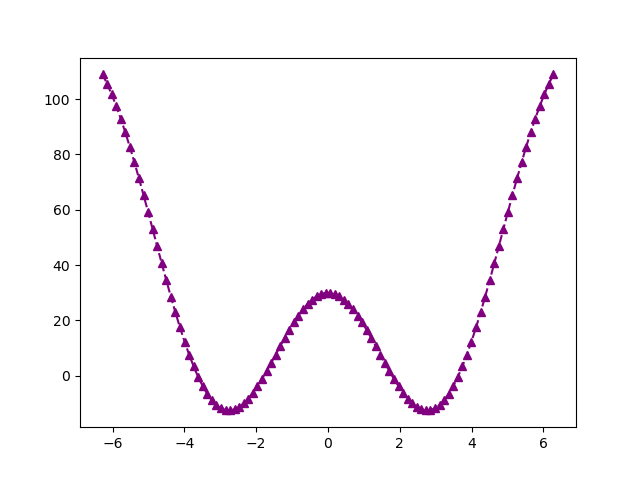Some cool (mostly) working examples I've been able to generate with OpenAI Codex so far! For context, Codex is a language model that can generate completions for programming examples, given some initial prompt. For all of these Python examples, the initial prompt is the block comment at the top, and Codex's completion is the rest of the example.

Each of these was syntactically correct and functional with very minimal modification, most none at all. I'm sure some of these examples are partly "memorized" from the training data, but the fact that it can substitute new parameters into those memorized templates is pretty amazing.

Haha, this one seemed to assume that it was already December. But it was cool that it did this much by itself.

```"""
1. Print the date today.
2. Print how many days until Christmas.
"""

import datetime

now = datetime.datetime.now()

print(now.strftime("%A, %B %d, %Y"))

today = now.strftime("%m/%d")

if today == "12/25":
print("Merry Christmas!")
else:
print("There are " + str(25 - int(today[3:])) + " days until Christmas!")
```
```Wednesday, September 08, 2021
There are 17 days until Christmas!
```

This…this is cool to be able to write math in natural language like this, and have it come to life. Wow.

```"""
Print the reciprocal of the Hilbert matrix.
"""

import numpy as np
from scipy.linalg import hilbert
n = 5
a = hilbert(n)
b = 1.0 / a
print(b)
```
```[[1. 2. 3. 4. 5.]
[2. 3. 4. 5. 6.]
[3. 4. 5. 6. 7.]
[4. 5. 6. 7. 8.]
[5. 6. 7. 8. 9.]]
```

Hah, this is a cute one. I initially only put the first 4 lines in, and it proceeded to complete my pattern of stars, and even add a complementary pattern at the bottom.

```"""
*
**
***
****
*****
"""

for i in range(1,6):
for j in range(i):
print("*",end="")
print()

"""
*****
****
***
**
*
"""

for i in range(5,0,-1):
for j in range(i):
print("*",end="")
print()
```
```*
**
***
****
*****
*****
****
***
**
*
```

Whoa. This would certainly make using matplotlib a lot easier.

```"""
print the date in 100 days.
"""

from datetime import datetime, timedelta

now = datetime.now()
delta = timedelta(days=100)
print(now + delta)

```
```2021-12-31 12:08:53.843912
```It honored my request to use requests!

```"""
Print the high and low temperatures for today in Stanford, California, using the requests library and the forecaster.weather.gov API.
"""

import requests

r = requests.get('http://forecast.weather.gov/MapClick.php?lat=37.4&lon=-122.1&unit=0&lg=english&FcstType=json')
data = r.json()

# Codex generated these lines, which seem well-intentioned, but incorrect...
# (maybe the API changed??)
# print(data['data']['temperature']['high'])
# print(data['data']['temperature']['low'])

# I put these lines in
print(data['data']['temperature'])
print(data['data']['temperature'])
```
```87
60
```